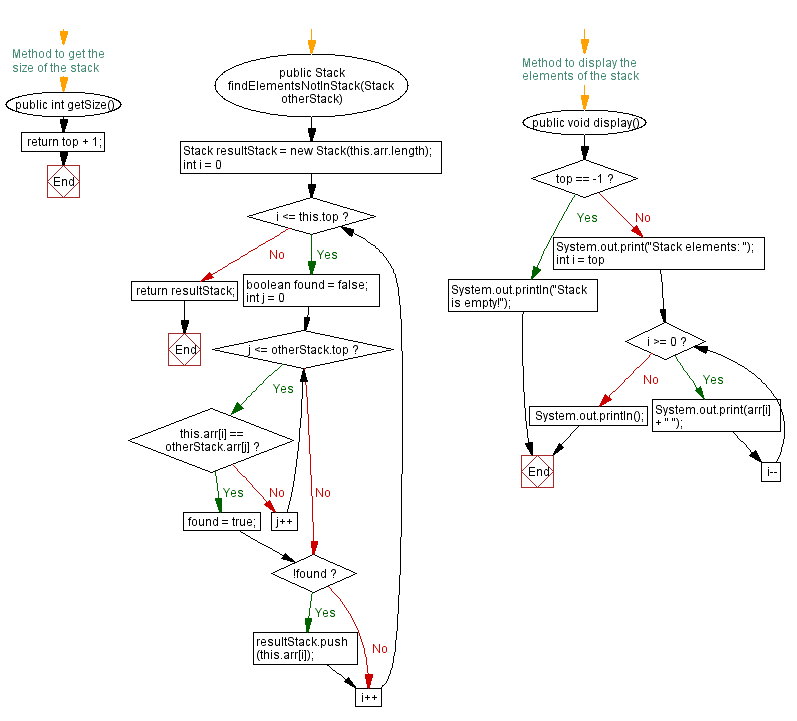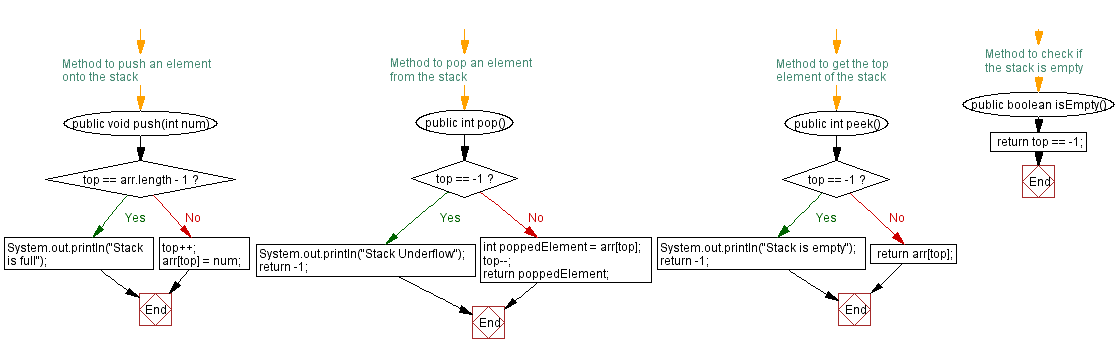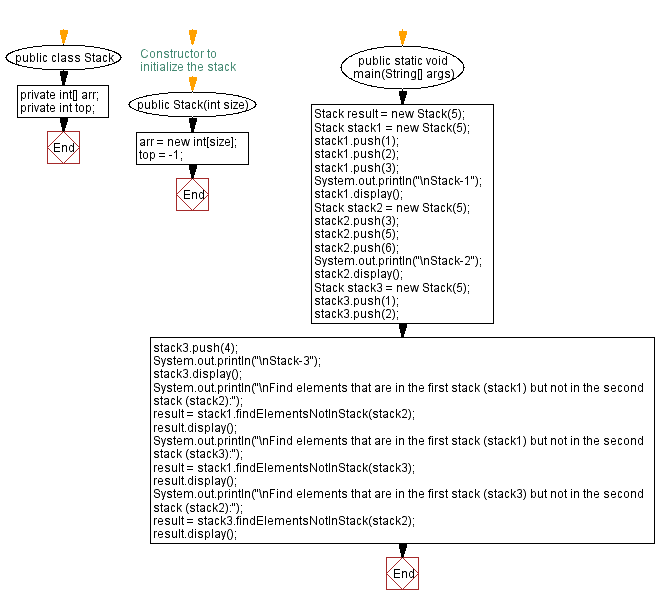# Java Exercises: Stack elements in the first but not in the second

## Java Stack: Exercise-22 with Solution

Write a Java program that implements a stack and find elements that are in the first stack but not in the second stack.

Sample Solution:

Java Code:

``````import java.util.Scanner;

public class Stack {
private int[] arr;
private int top;

// Constructor to initialize the stack
public Stack(int size) {
arr = new int[size];
top = -1;
}

// Method to push an element onto the stack
public void push(int num) {
if (top == arr.length - 1) {
System.out.println("Stack is full");
} else {
top++;
arr[top] = num;
}
}

// Method to pop an element from the stack
public int pop() {
if (top == -1) {
System.out.println("Stack Underflow");
return -1;
} else {
int poppedElement = arr[top];
top--;
return poppedElement;
}
}

// Method to get the top element of the stack
public int peek() {
if (top == -1) {
System.out.println("Stack is empty");
return -1;
} else {
return arr[top];
}
}

// Method to check if the stack is empty
public boolean isEmpty() {
}

// Method to get the size of the stack
public int getSize() {
}

public Stack findElementsNotInStack(Stack otherStack) {
Stack resultStack = new Stack(this.arr.length);
for (int i = 0; i <= this.top; i++) {
boolean found = false;
for (int j = 0; j <= otherStack.top; j++) {
if (this.arr[i] == otherStack.arr[j]) {
found = true;
break;
}
}
if (!found) {
resultStack.push(this.arr[i]);
}
}
return resultStack;
}
// Method to display the elements of the stack
public void display() {
if (top == -1) {
System.out.println("Stack is empty!");
} else {
System.out.print("Stack elements: ");
for (int i = top; i >= 0; i--) {
System.out.print(arr[i] + " ");
}
System.out.println();
}
}

public static void main(String[] args) {
Stack result = new Stack(5);
Stack stack1 = new Stack(5);
stack1.push(1);
stack1.push(2);
stack1.push(3);
System.out.println("\nStack-1");
stack1.display();
Stack stack2 = new Stack(5);
stack2.push(3);
stack2.push(5);
stack2.push(6);
System.out.println("\nStack-2");
stack2.display();
Stack stack3 = new Stack(5);
stack3.push(1);
stack3.push(2);
stack3.push(4);
System.out.println("\nStack-3");
stack3.display();
System.out.println("\nFind elements that are in the first stack (stack1) but not in the second stack (stack2):");
result = stack1.findElementsNotInStack(stack2);
result.display();
System.out.println("\nFind elements that are in the first stack (stack1) but not in the second stack (stack3):");
result = stack1.findElementsNotInStack(stack3);
result.display();
System.out.println("\nFind elements that are in the first stack (stack3) but not in the second stack (stack2):");
result = stack3.findElementsNotInStack(stack2);
result.display();
}
}
```
```

Sample Output:

```Stack-1
Stack elements: 3 2 1

Stack-2
Stack elements: 6 5 3

Stack-3
Stack elements: 4 2 1

Find elements that are in the first stack (stack1) but not in the second stack (stack2):
Stack elements: 2 1

Find elements that are in the first stack (stack1) but not in the second stack (stack3):
Stack elements: 3

Find elements that are in the first stack (stack3) but not in the second stack (stack2):
Stack elements: 4 2 1
```

Flowchart:Live Demo:

Java Code Editor:

Improve this sample solution and post your code through Disqus

Java Stack Previous: Find common elements between two stacks.
Java Stack Exercises Next: Elements from two stacks without duplicates.

What is the difficulty level of this exercise?

Test your Programming skills with w3resource's quiz.

﻿